# What is Python Dictionary and How to Use It

Python dictionary is an unordered collection of elements that are changeable and indexed. Dictionaries are written with curly braces, and they have keys and values. While other compound data types like lists or tuples have only value as an item, a dictionary has a key-value pair.

## How to create a dictionary in Python

To create a dictionary in Python, place elements inside curly braces {} separated by a comma. An element has a key, and the corresponding value is expressed as a key-value pair.

Let us create a simple Dictionary in Python.

```# app.py

dictA = {'name': 'Krunal', 'age': 26}
print(dictA)```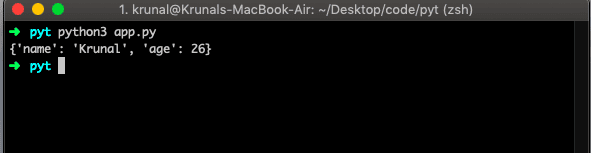The above is the simplest way to create a dictionary.

In Dictionary, the values can be of any data type and can repeat, but the keys must be immutable and unique. Therefore, dictionaries must have unique keys.

We can also use the dict() method to create a dictionary.

```# app.py

print(dictB)```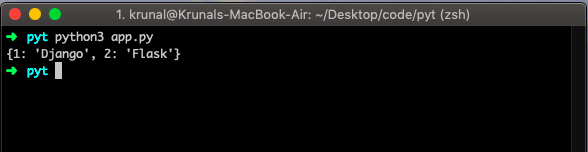## How to access elements in Dictionary

To access elements in the Python dictionary, refer to their key name inside square brackets. See the below example.

```# app.py

print(dictB)```

See the output below.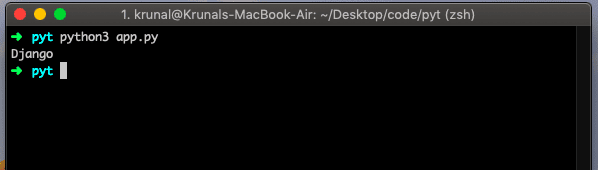You can also use the method called get(), which will give you the same result.

```dictB = dict({1:'Django', 2:'Flask'})
print(dictB.get(1))```

## How to change in Dictionary in Python

To change a dictionary in Python, use an assignment operator.

A dictionary is mutable; that is why we can add new items or change the value of an existing object. In addition, you can change the value of the specific element by referring to its key name.

If the key is already present, the value gets updated; a new key: value pair is added to the Dictionary.

Let’s see the below example.

```# app.py

dictC = {'phpf1': 'Laravel', 'phpf2':'Symfony', 'phpf3':'Zend'}
print(dictC)
dictC['phpf3'] = 'Yii'
print('After changing values: ')
print(dictC)```

See the output below.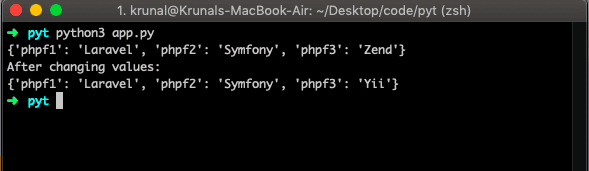Add the item to the Dictionary.

```# app.py

dictC = {'phpf1': 'Laravel', 'phpf2':'Symfony', 'phpf3':'Zend'}
print(dictC)
dictC['phpf4'] = 'Yii'
print(dictC)```

See the output.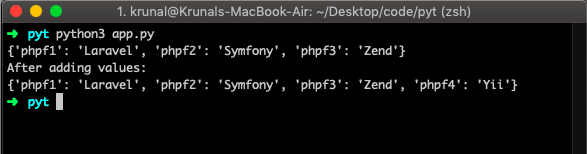## How to remove an element from a dictionary

To remove an element from a dictionary in Python, use the dict.pop() method.  The pop() method removes an item with the provided key and returns the value.

See the below example.

```# app.py

dictD = {1:18, 2:19, 3:20, 4:21}
poppedE = dictD.pop(3)
print('Popped Item: ',poppedE)
print(dictD)```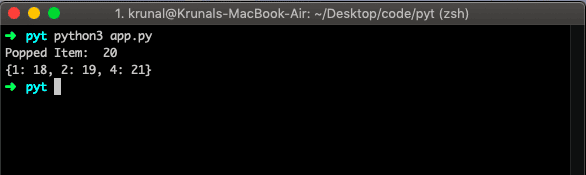## How to loop through a Dictionary in Python

To loop through a dictionary in Python, use the for loop. The for loop is used to iterate each element of the Dictionary. When looping through the Dictionary, the return value is the key to the Dictionary, but we can also return the values.

Let us print the values of the Dictionary.

```# app.py

dictK = {'c1': 'Ben 10', 'c2': 'Kung Fu Panda', 'c3': 'Scooby Doo'}
for key in dictK:
print(dictK[key])```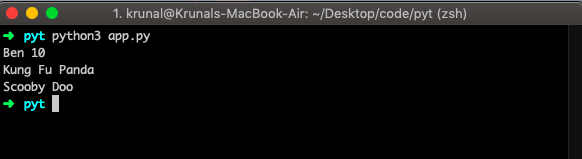We can also print the keys. See the below code.

```# app.py

dictK = {'c1': 'Ben 10', 'c2': 'Kung Fu Panda', 'c3': 'Scooby Doo'}
for key in dictK:
print(key)```

## Dictionary Length

To find a length of a dictionary in Python, use the len() method.

```# app.py

dictC = {'phpf1': 'Laravel', 'phpf2':'Symfony', 'phpf3':'Zend'}
print(len(dictC))```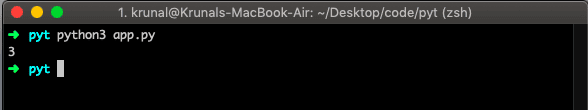That’s it for this tutorial.

This site uses Akismet to reduce spam. Learn how your comment data is processed.